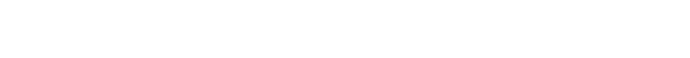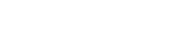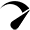# Predictable Projectile In Unity

I recently started working on a top-down shooter game where the enemies want to shoot or blow the player and I made a simple firing script that just adds a force towards the direction of the player with a constant speed, and this made the enemies look considerably dumb because they kept on missing once the player makes a move and this is because they are shooting at the player current position and when the projectile gets to the player he has already moved. So I decided to look up how predictive firing works or a simple algorithm that would help me implement it. I found a few good sources but non had a simple code implementation and I am not the best mathematician so I decided to break down what I had gotten from various sources to its simplest form.

Note: I highly recommend you read the references before going on as they are some things I didn’t note down for simplicity’s sake. The references are at the end of the page.

## The Obstacle I Faced

Aim and fire a projectile at a moving target with a constant speed.

## Predicting Player Position

Let’s begin with predicting where the player will be.

In this implementation, there will be no rotation, and the player’s vertical position will not be taken into account as there no jumping in my project.
We need to know which direction is player is going and we can get that by knowing how fast the player is going at that moment, so we get the player’s velocity.

In Unity, you can get your targets/players velocity by using a history buffer or by just adding a Rigidbody and getting the velocity direction from the Rigidbody.

We also need a time value to know how much time the player will be moving for.The formula gives us our predicted position vector Np.

## Firing Linear Projectile

This is a linear projectile so no gravity is applied, I will skip any line of sight check or firing delays since this is a very simple implementation but you can easily add them in code.

First, we would need the initial position of the projectile, which in most cases is the muzzle of a gun

We also need a constant projectile speed and also the time value for the amount of time it runs.This can also be written as the equation of a circle, like thisSo now we have both equations for predicted player movement and projectile position, if you look at the equations the Np is our intersection point on the circle, we don’t know t which is our intersection time.

if we solve these equations, we would end up with a quadratic equation which we can use to solve for t.Reminder: a, b and c are scalars, if you want to know how the derivation came about check the reference below, it’s all detailed out.

There are a few special cases. If the discriminant is negative then no solution but if the discriminant is 0, we have a solution.

## Code

First, create a Method that has parameters for

• PlayerPosition
• PlayerVelocity
• ProjectileLaunchPos
• ProjectileSpeed
• TimeMy method will return a bool to check if the solution is valid so I created a temporary bool called “valid”

then I take the direction from the ProjectileLaunchPos to the PlayerPosition and set the y value to 0, I also set the PlayerVelocityValue to 0 just in case.Then I solve for a, b, and c using the dot product for the vector multiplicationThen I solve the determinant and make sure the methods only return true when the determinant is > 0 and with a quadratic equation you always get two solutions so I pick the solution with the max value and I just do a simple debug to know when I get a determinant < 0After the calculations, I just return the time value and return true if valid.

If you get a valid solution then all you need is to plug the t value into the two solutions and you get a vector that fires at a predicted solution.

## SOCIAL NETWORKSOptimized by Seraphinite Accelerator